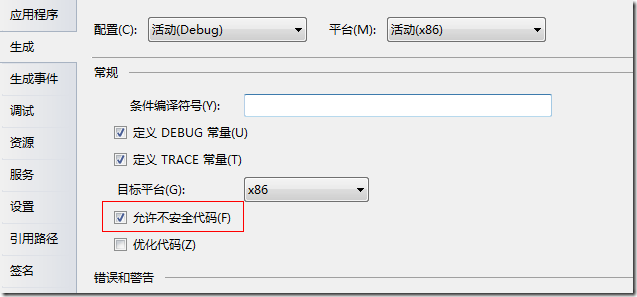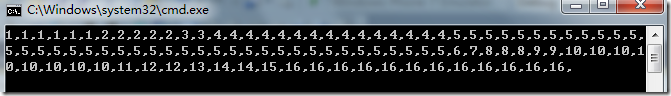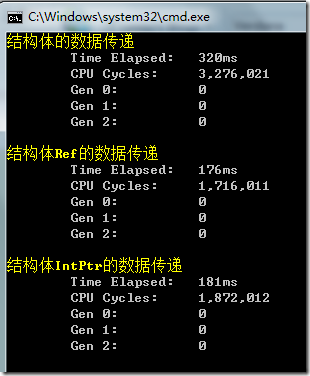# 对于.Net中C#指针的研究

在C/C++中，对于指针的使用是很普遍的，可以这么说，如果没有指针的运用，都不知道程序如何来写。在.Net中，同样也是可以使用指针的，不过必须通过开启不安全的代码来使用。在默认情况下，新建的项目是不允许使用不安全的代码，这样，就必须通过设置项目来开启使用，通过设置项目“属性”的“生成”来达到：1、unsafe

unsafe static void Main(string[] args)

// 不安全代码编写

}

unsafe
{

// 不安全代码编写

}

2、stackalloc

关键字stackalloc是用于申请栈内存。stackalloc类似于C库中的_alloca。通过使用stackalloc可以自动启用CLR中的缓冲区溢出检测功能。当函数执行完毕后，内存会被自动回收。

使用它的目的通过它来实现对栈内存的使用，从而减少对堆的使用，减少系统开销。

int* array = stackalloc int;
for (int index = 0; index < 10; index++

array[index]
= index;

for (int index = 0; index < 10; index++

Console.WriteLine(array[index].ToString());

3、IntPtr 和 ref

IntPtr是.Net提供的托管指针，ref是C#语言中的关键字，和IntPtr没有关系，尽管它们使用起来很类似。区别在于，使用ref可以引用Class，共同点它们都可以引用值类型，包括Struct结构体等等。

[StructLayout(LayoutKind.Sequential)]

public struct ST_TERMPARA
{
[MarshalAs(UnmanagedType.ByValArray, SizeConst
= 6)]

public byte[] szAcqID_n_9F01;                /**<(TERM)收单行标识*/
[MarshalAs(UnmanagedType.ByValArray, SizeConst
= 5)]

public byte[] szAddTermCap_b_9F40;        /**<(TERM)附加终端性能*/
[MarshalAs(UnmanagedType.ByValArray, SizeConst
= 2)]

public byte[] szMerCateCode_n_9F15;        /**<(TERM)商户分类码*/
[MarshalAs(UnmanagedType.ByValArray, SizeConst
= 15)]

public byte[] szMerID_ans_9F16;            /**<(TERM)商户标识*/
[MarshalAs(UnmanagedType.ByValArray, SizeConst
= 40)]

public byte[] szMerNameLoc_ans;            /**<(TERM)商户名称和位置*/

public byte cMessageType_n;                /**<(TERM)报文类别*/

public byte cEntryMode_n_9F39;                /**<(TERM)销售点（POS）输入方式*/
[MarshalAs(UnmanagedType.ByValArray, SizeConst
= 3)]

public byte[] szTermCap_b_9F33;            /**<(TERM)终端性能*/
[MarshalAs(UnmanagedType.ByValArray, SizeConst
= 2)]

public byte[] szTermCountryCode_b_9F1A;    /**<(TERM)终端国家代码*/
[MarshalAs(UnmanagedType.ByValArray, SizeConst
= 8)]

public byte[] szTermId_an_9F1C;            /**<(TERM)终端号*/

public byte cTypeTerm_n_9F35;                /**<(TERM)终端类型*/
[MarshalAs(UnmanagedType.ByValArray, SizeConst
= 2)]

public byte[] szCurCode_n_5F2A;            /**<(TERM)交易货币代码*/

public byte cCurExp_n_5F36;                /**<(TERM)交易货币指数*/
[MarshalAs(UnmanagedType.ByValArray, SizeConst
= 2)]

public byte[] szRefCurrCode_n_9F3C;        /**<(TERM)交易参考货币代码*/

public byte cRefCurrExp_n_9F3D;            /**<(TERM)交易参考货币指数*/
[MarshalAs(UnmanagedType.ByValArray, SizeConst
= 4)]

public byte[] szRefRate_n;                    /**<(TERM)交易参考货币兑换比率*/
[MarshalAs(UnmanagedType.ByValArray, SizeConst
= 8)]

public byte[] szIFD_an_9F1E;                /**<(TERM)接口设备（IFD）序列号*/
}

ST_TERMPARA para;
para.szAcqID_n_9F01
= Enumerable.Repeat<byte>(0x016).ToArray();
para.szAddTermCap_b_9F40
= Enumerable.Repeat<byte>(0x025).ToArray();
para.szMerCateCode_n_9F15
= Enumerable.Repeat<byte>(0x032).ToArray();
para.szMerID_ans_9F16
= Enumerable.Repeat<byte>(0x0415).ToArray();
para.szMerNameLoc_ans
= Enumerable.Repeat<byte>(0x0540).ToArray();
para.cMessageType_n
= 0x06
para.cEntryMode_n_9F39
= 0x07
para.szTermCap_b_9F33
= Enumerable.Repeat<byte>(0x083).ToArray();
para.szTermCountryCode_b_9F1A
= Enumerable.Repeat<byte>(0x092).ToArray();
para.szTermId_an_9F1C
= Enumerable.Repeat<byte>(0x0a8).ToArray();
para.cTypeTerm_n_9F35
= 0x0b
para.szCurCode_n_5F2A
= Enumerable.Repeat<byte>(0x0c2).ToArray();
para.cCurExp_n_5F36
= 0x0d
para.szRefCurrCode_n_9F3C
= Enumerable.Repeat<byte>(0x0e2).ToArray();
para.cRefCurrExp_n_9F3D
= 0x0f
para.szRefRate_n
= Enumerable.Repeat<byte>(0x104).ToArray();
para.szIFD_an_9F1E
= Enumerable.Repeat<byte>(0x108).ToArray();

int size = Marshal.SizeOf(para);
IntPtr intPtr
= Marshal.AllocHGlobal(size);
Marshal.StructureToPtr(para, intPtr,
true);
byte* bytes = (byte*)intPtr.ToPointer();

for (int index = 0; index < size; index++

Console.Write(
*(bytes + index) + ",");CodeTimer.Time("结构体的数据传递"10000000, () => { GetValue(para); });

CodeTimer.Time(
"结构体Ref的数据传递"10000000, () => { GetValue(ref para); });

CodeTimer.Time(
"结构体IntPtr的数据传递"10000000, () => { GetValue(intPtr); });

static void GetValue(ST_TERMPARA para)

// ...
}

static void GetValue(ref ST_TERMPARA para)

// ...
}

static void GetValue(IntPtr intPtr)

// ...posted @ 2011-07-28 11:55  Leepy  阅读(10168)  评论(19编辑  收藏  举报